# RD Sharma Solutions for Class 8 Maths Chapter 14 Compound Interest Exercise 14.1

In Exercise 14.1 of Chapter 14, we shall discuss problems based on the computation of compound interest when interest is compounded annually, half-yearly and quarterly. Students can refer to RD Sharma Solutions which is designed by our expert tutors for the purpose of strengthening the concepts and problem-solving abilities. Solutions here are developed with utmost care to help students analyze the problems competently and hence score good marks in their exams. Students can download the pdf from the links provided below.

## Download the Pdf of RD Sharma Solutions for Class 8 Maths Exercise 14.1 Chapter 14 Compound Interest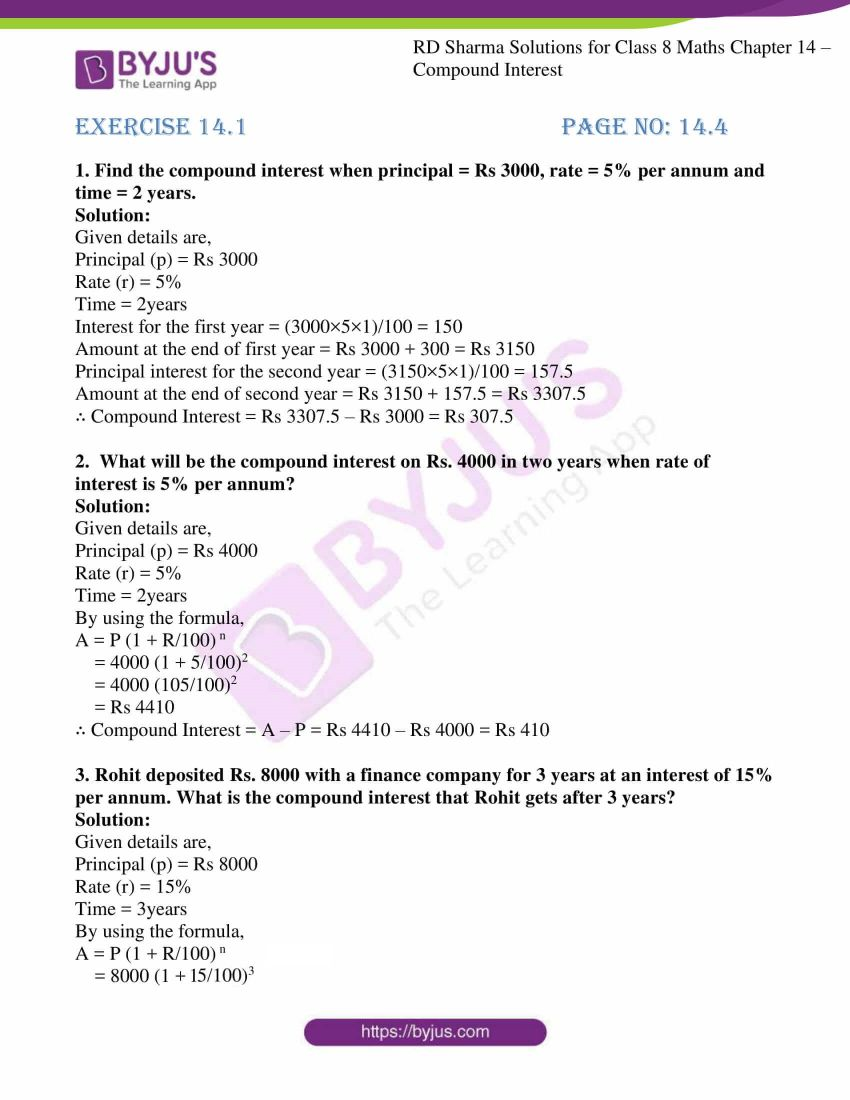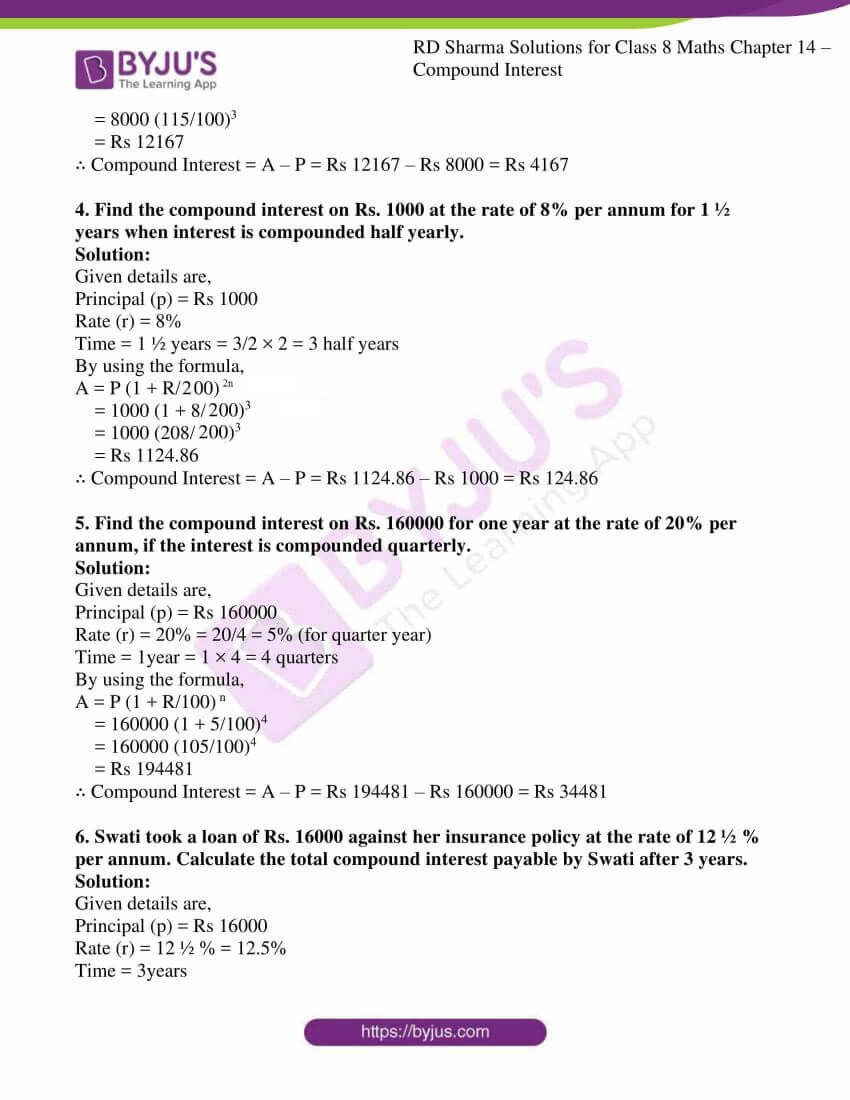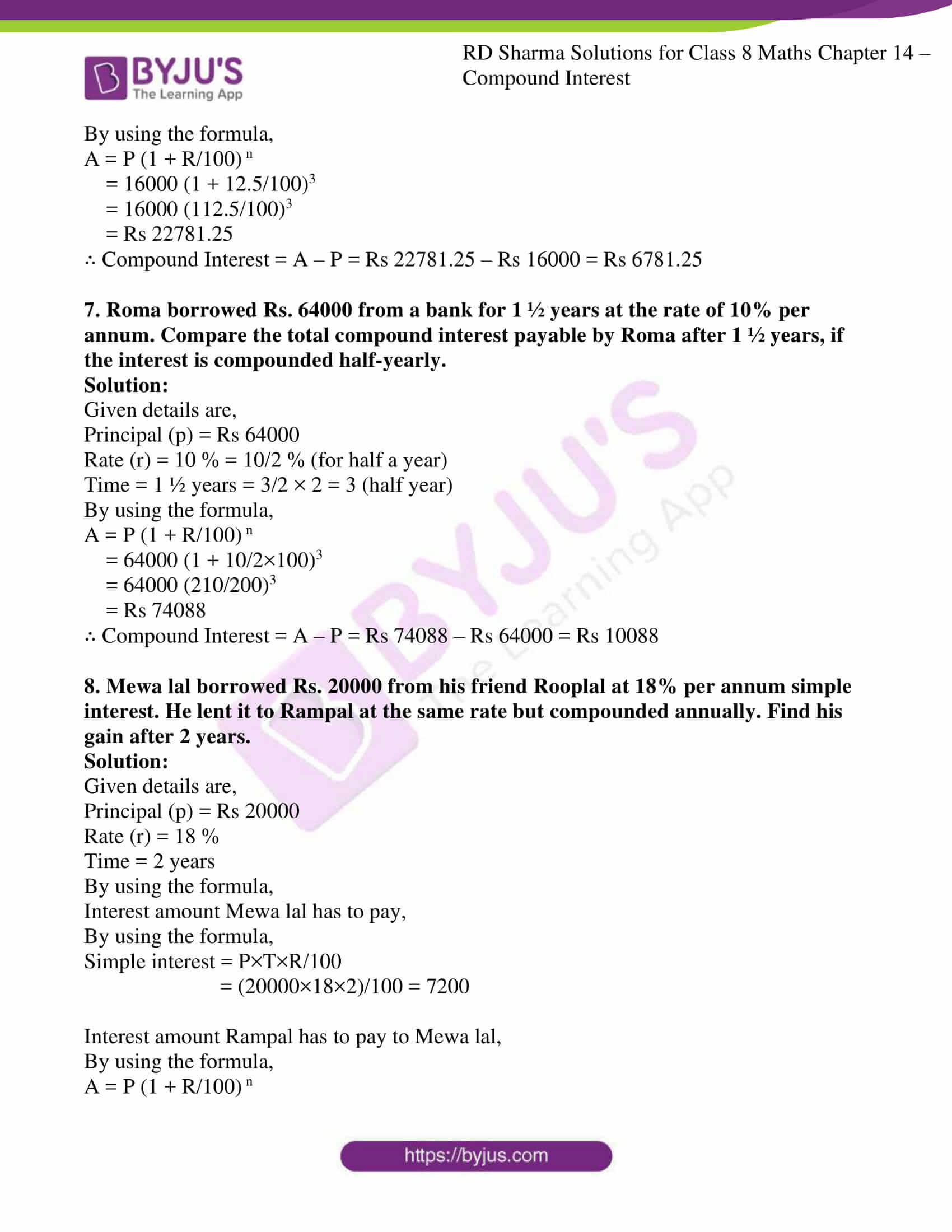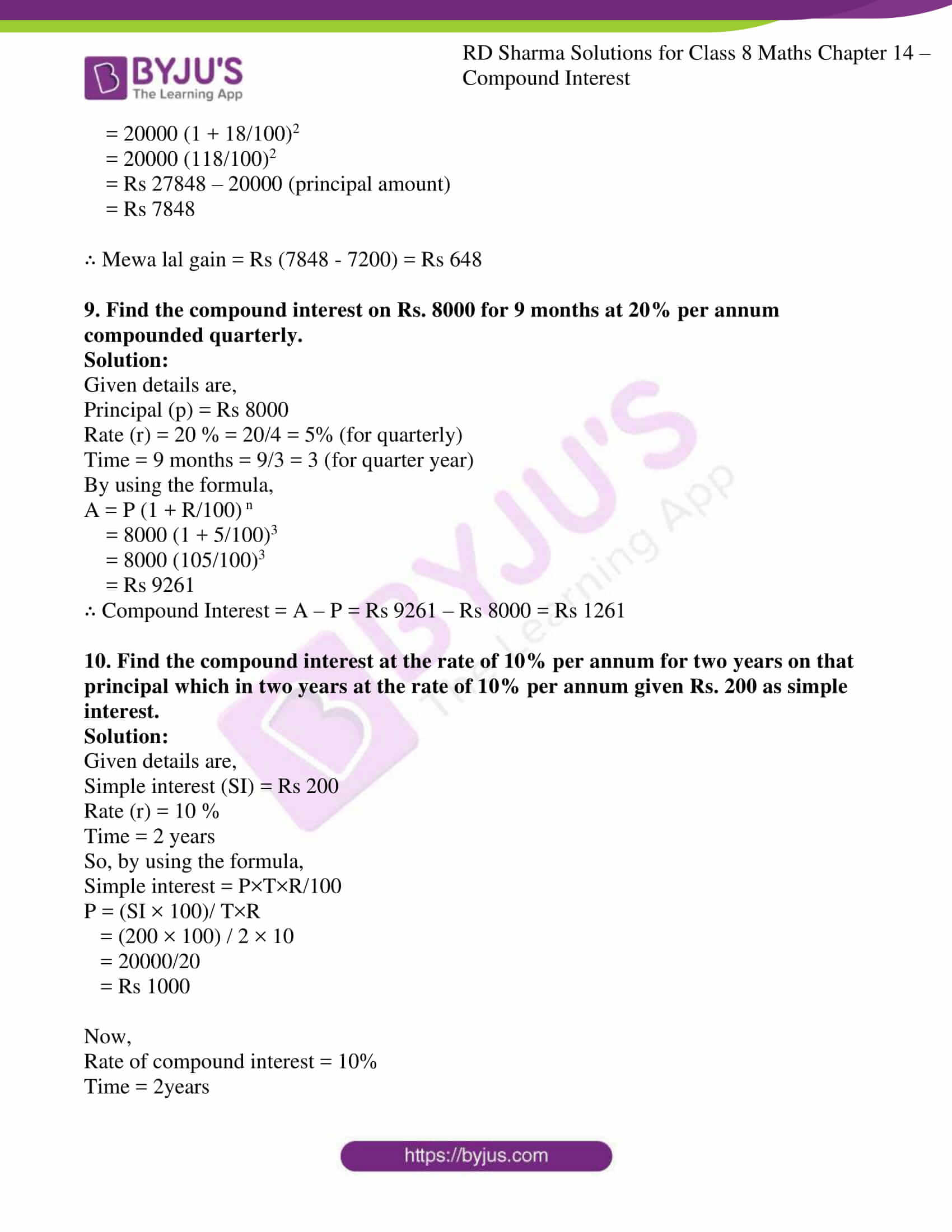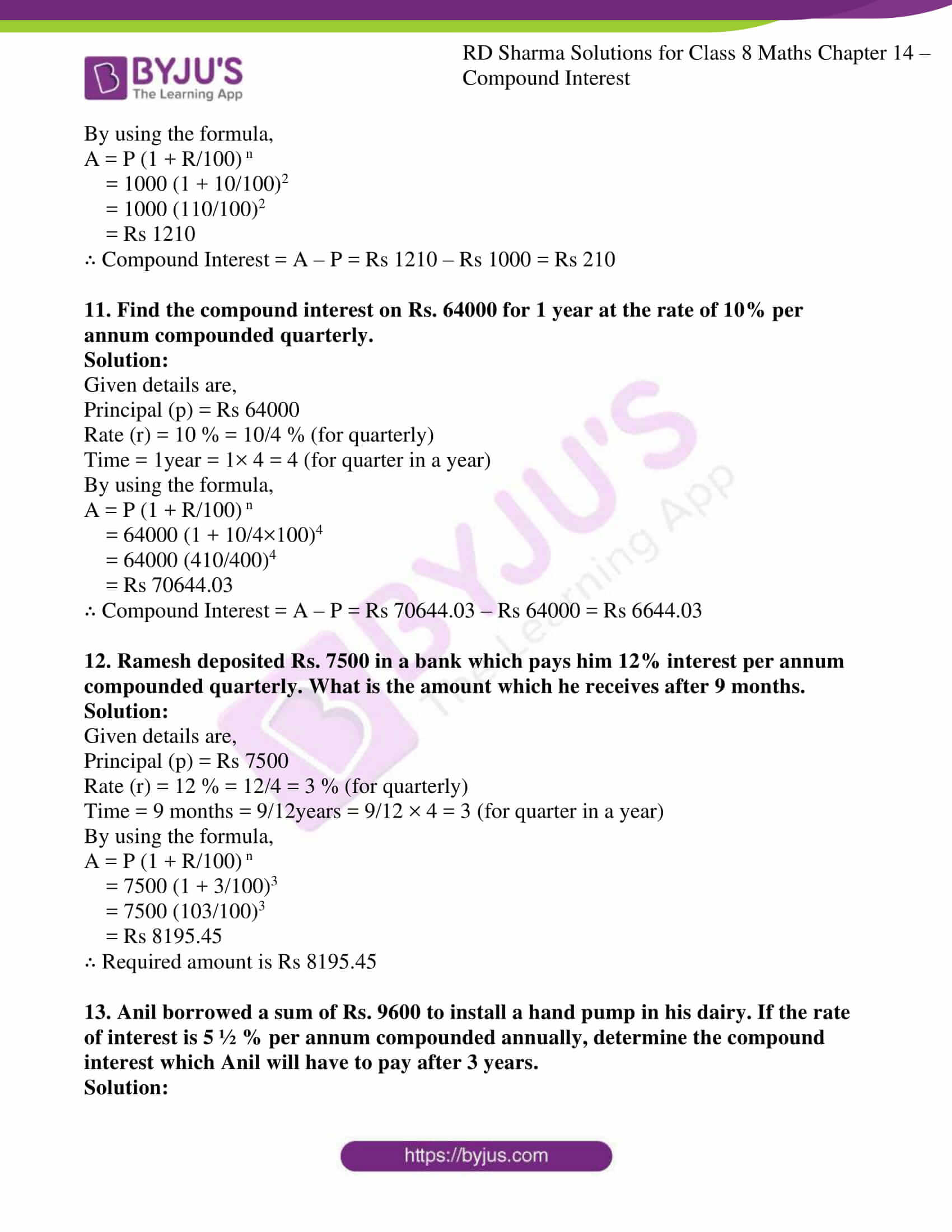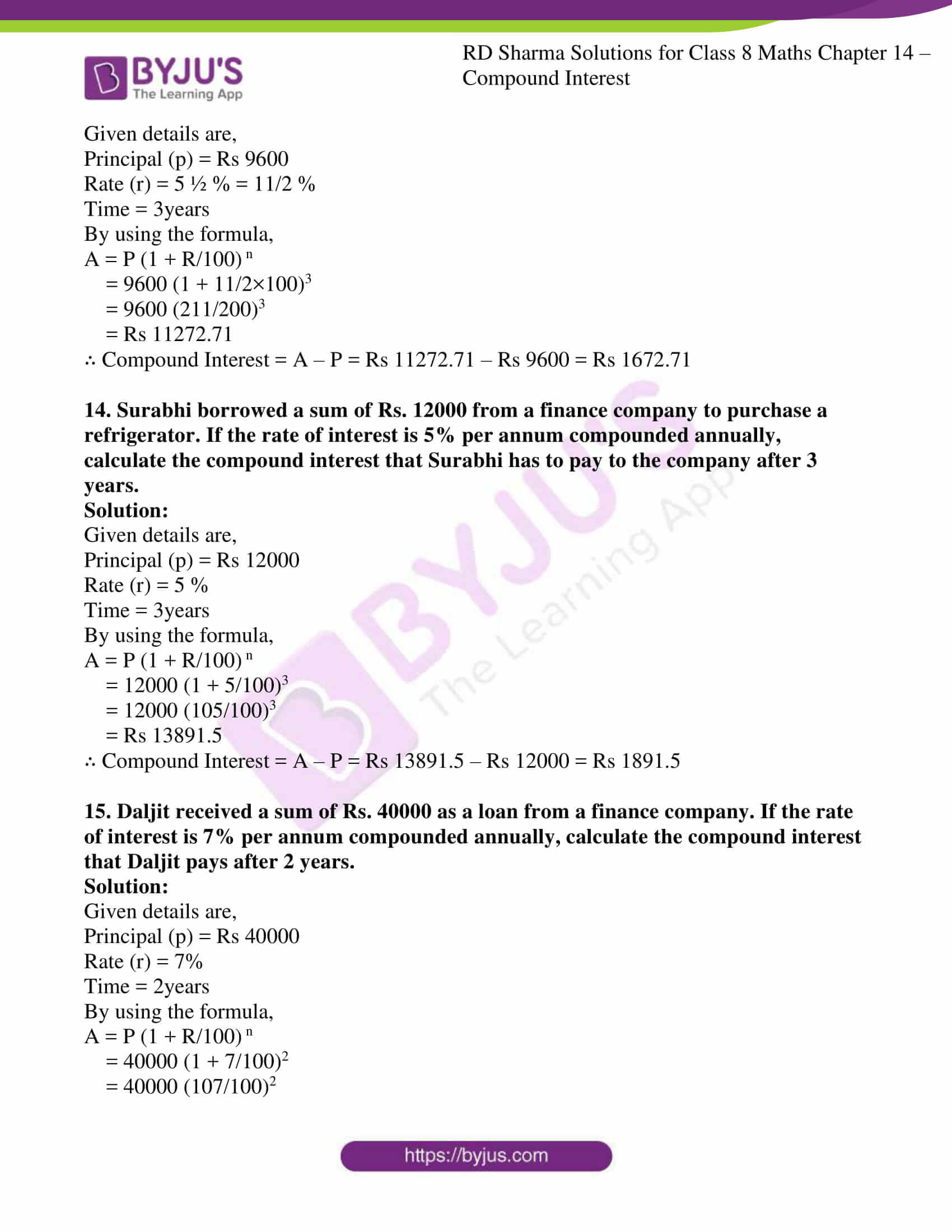### Access other exercises of RD Sharma Solutions for Class 8 Maths Chapter 14 Compound Interest

Exercise 14.2 Solutions

Exercise 14.3 Solutions

Exercise 14.4 Solutions

Exercise 14.5 Solutions

### Access answers to Maths RD Sharma Solutions For Class 8 Exercise 14.1 Chapter 14 Compound Interest

1. Find the compound interest when principal = Rs 3000, rate = 5% per annum and time = 2 years.

Solution:

Given details are,

Principal (p) = Rs 3000

Rate (r) = 5%

Time = 2years

Interest for the first year = (3000×5×1)/100 = 150

Amount at the end of first year = Rs 3000 + 300 = Rs 3150

Principal interest for the second year = (3150×5×1)/100 = 157.5

Amount at the end of second year = Rs 3150 + 157.5 = Rs 3307.5

∴ Compound Interest = Rs 3307.5 – Rs 3000 = Rs 307.5

2. What will be the compound interest on Rs. 4000 in two years when rate of interest is 5% per annum?

Solution:

Given details are,

Principal (p) = Rs 4000

Rate (r) = 5%

Time = 2years

By using the formula,

A = P (1 + R/100) n

= 4000 (1 + 5/100)2

= 4000 (105/100)2

= Rs 4410

∴ Compound Interest = A – P = Rs 4410 – Rs 4000 = Rs 410

3. Rohit deposited Rs. 8000 with a finance company for 3 years at an interest of 15% per annum. What is the compound interest that Rohit gets after 3 years?

Solution:

Given details are,

Principal (p) = Rs 8000

Rate (r) = 15%

Time = 3years

By using the formula,

A = P (1 + R/100) n

= 8000 (1 + 15/100)3

= 8000 (115/100)3

= Rs 12167

∴ Compound Interest = A – P = Rs 12167 – Rs 8000 = Rs 4167

4. Find the compound interest on Rs. 1000 at the rate of 8% per annum for 1 ½ years when interest is compounded half yearly.

Solution:

Given details are,

Principal (p) = Rs 1000

Rate (r) = 8%

Time = 1 ½ years = 3/2 × 2 = 3 half years

By using the formula,

A = P (1 + R/200) 2n

= 1000 (1 + 8/200)3

= 1000 (208/200)3

= Rs 1124.86

∴ Compound Interest = A – P = Rs 1124.86 – Rs 1000 = Rs 124.86

5. Find the compound interest on Rs. 160000 for one year at the rate of 20% per annum, if the interest is compounded quarterly.

Solution:

Given details are,

Principal (p) = Rs 160000

Rate (r) = 20% = 20/4 = 5% (for quarter year)

Time = 1year = 1 × 4 = 4 quarters

By using the formula,

A = P (1 + R/100) n

= 160000 (1 + 5/100)4

= 160000 (105/100)4

= Rs 194481

∴ Compound Interest = A – P = Rs 194481 – Rs 160000 = Rs 34481

6. Swati took a loan of Rs. 16000 against her insurance policy at the rate of 12 ½ % per annum. Calculate the total compound interest payable by Swati after 3 years.

Solution:

Given details are,

Principal (p) = Rs 16000

Rate (r) = 12 ½ % = 12.5%

Time = 3years

By using the formula,

A = P (1 + R/100) n

= 16000 (1 + 12.5/100)3

= 16000 (112.5/100)3

= Rs 22781.25

∴ Compound Interest = A – P = Rs 22781.25 – Rs 16000 = Rs 6781.25

7. Roma borrowed Rs. 64000 from a bank for 1 ½ years at the rate of 10% per annum. Compare the total compound interest payable by Roma after 1 ½ years, if the interest is compounded half-yearly.

Solution:

Given details are,

Principal (p) = Rs 64000

Rate (r) = 10 % = 10/2 % (for half a year)

Time = 1 ½ years = 3/2 × 2 = 3 (half year)

By using the formula,

A = P (1 + R/100) n

= 64000 (1 + 10/2×100)3

= 64000 (210/200)3

= Rs 74088

∴ Compound Interest = A – P = Rs 74088 – Rs 64000 = Rs 10088

8. Mewa lal borrowed Rs. 20000 from his friend Rooplal at 18% per annum simple interest. He lent it to Rampal at the same rate but compounded annually. Find his gain after 2 years.

Solution:

Given details are,

Principal (p) = Rs 20000

Rate (r) = 18 %

Time = 2 years

By using the formula,

Interest amount Mewa lal has to pay,

By using the formula,

Simple interest = P×T×R/100

= (20000×18×2)/100 = 7200

Interest amount Rampal has to pay to Mewa lal,

By using the formula,

A = P (1 + R/100) n

= 20000 (1 + 18/100)2

= 20000 (118/100)2

= Rs 27848 – 20000 (principal amount)

= Rs 7848

∴ Mewa lal gain = Rs (7848 – 7200) = Rs 648

9. Find the compound interest on Rs. 8000 for 9 months at 20% per annum compounded quarterly.

Solution:

Given details are,

Principal (p) = Rs 8000

Rate (r) = 20 % = 20/4 = 5% (for quarterly)

Time = 9 months = 9/3 = 3 (for quarter year)

By using the formula,

A = P (1 + R/100) n

= 8000 (1 + 5/100)3

= 8000 (105/100)3

= Rs 9261

∴ Compound Interest = A – P = Rs 9261 – Rs 8000 = Rs 1261

10. Find the compound interest at the rate of 10% per annum for two years on that principal which in two years at the rate of 10% per annum given Rs. 200 as simple interest.

Solution:

Given details are,

Simple interest (SI) = Rs 200

Rate (r) = 10 %

Time = 2 years

So, by using the formula,

Simple interest = P×T×R/100

P = (SI × 100)/ T×R

= (200 × 100) / 2 × 10

= 20000/20

= Rs 1000

Now,

Rate of compound interest = 10%

Time = 2years

By using the formula,

A = P (1 + R/100) n

= 1000 (1 + 10/100)2

= 1000 (110/100)2

= Rs 1210

∴ Compound Interest = A – P = Rs 1210 – Rs 1000 = Rs 210

11. Find the compound interest on Rs. 64000 for 1 year at the rate of 10% per annum compounded quarterly.

Solution:

Given details are,

Principal (p) = Rs 64000

Rate (r) = 10 % = 10/4 % (for quarterly)

Time = 1year = 1× 4 = 4 (for quarter in a year)

By using the formula,

A = P (1 + R/100) n

= 64000 (1 + 10/4×100)4

= 64000 (410/400)4

= Rs 70644.03

∴ Compound Interest = A – P = Rs 70644.03 – Rs 64000 = Rs 6644.03

12. Ramesh deposited Rs. 7500 in a bank which pays him 12% interest per annum compounded quarterly. What is the amount which he receives after 9 months.

Solution:

Given details are,

Principal (p) = Rs 7500

Rate (r) = 12 % = 12/4 = 3 % (for quarterly)

Time = 9 months = 9/12years = 9/12 × 4 = 3 (for quarter in a year)

By using the formula,

A = P (1 + R/100) n

= 7500 (1 + 3/100)3

= 7500 (103/100)3

= Rs 8195.45

∴ Required amount is Rs 8195.45

13. Anil borrowed a sum of Rs. 9600 to install a hand pump in his dairy. If the rate of interest is 5 ½ % per annum compounded annually, determine the compound interest which Anil will have to pay after 3 years.

Solution:

Given details are,

Principal (p) = Rs 9600

Rate (r) = 5 ½ % = 11/2 %

Time = 3years

By using the formula,

A = P (1 + R/100) n

= 9600 (1 + 11/2×100)3

= 9600 (211/200)3

= Rs 11272.71

∴ Compound Interest = A – P = Rs 11272.71 – Rs 9600 = Rs 1672.71

14. Surabhi borrowed a sum of Rs. 12000 from a finance company to purchase a refrigerator. If the rate of interest is 5% per annum compounded annually, calculate the compound interest that Surabhi has to pay to the company after 3 years.

Solution:

Given details are,

Principal (p) = Rs 12000

Rate (r) = 5 %

Time = 3years

By using the formula,

A = P (1 + R/100) n

= 12000 (1 + 5/100)3

= 12000 (105/100)3

= Rs 13891.5

∴ Compound Interest = A – P = Rs 13891.5 – Rs 12000 = Rs 1891.5

15. Daljit received a sum of Rs. 40000 as a loan from a finance company. If the rate of interest is 7% per annum compounded annually, calculate the compound interest that Daljit pays after 2 years.

Solution:

Given details are,

Principal (p) = Rs 40000

Rate (r) = 7%

Time = 2years

By using the formula,

A = P (1 + R/100) n

= 40000 (1 + 7/100)2

= 40000 (107/100)2

= Rs 45796

∴ Compound Interest = A – P = Rs 45796 – Rs 40000 = Rs 5796